# About

Esfandiar Manii - CSC 572 - Spring 2012

The current project focuses on designing a method to simulate explosions in 3d for game developing purposes.

# Modeling

Representation of explosions is a heavy process for the CPU to perform. Although there are many models to represent explosions in the 3d space, for real-time processing such as games, it is required to implement a simple and light weight method to perform this operation. In this project, explosions are simulated by using a physical modeling represented by kinematic equations.

Any volume can be represented using triangles. Each triangle consists of four components: Vertices, a Normal Vector, a Color Vector, and a Force Vector. The first three components are designed based on the mesh characteristics. The last component, the force, must be defined via two other components: Direction and Force magnitude. To calculate the force direction, it can be assumed that the force direction is perpendicular to the surfaces. This force represents the inter-component mutual force between two triangles. The force magnitude can be determined manually by visual tests.

A triangle is represented by its vertices and normal in 3d space. Therefore to calculate the force direction (as our assumption), it is required to calculate the vector which is perpendicular to the normal of the triangle.
As is presented in the figure below, the normal of each triangle and the force vectors are provided in black and red respectively.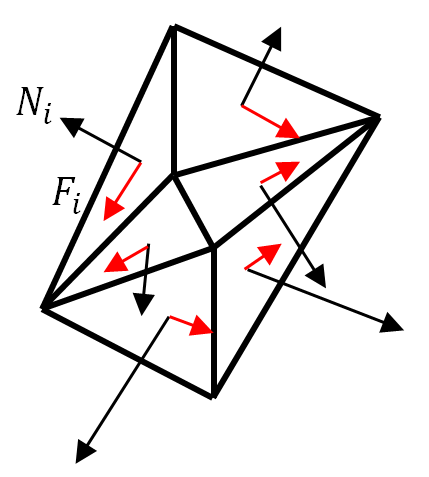To formulate this relation we can use: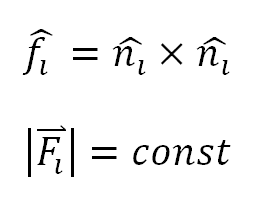When the player shoots the object in the front of them, the direction vector of aiming and the shooting power can be formulized by: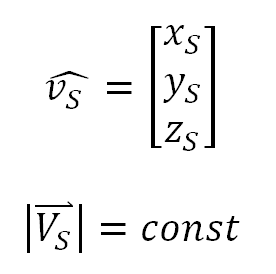Finally, the resultant vector, which sums the shooting vector and inter-component force vector, can be calculated by: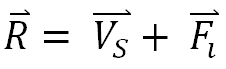By having the Kinematic Equations, the applied acceleration to each triangle can be calculated by: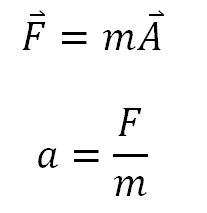Therefore, the acceleration vector can be represented by: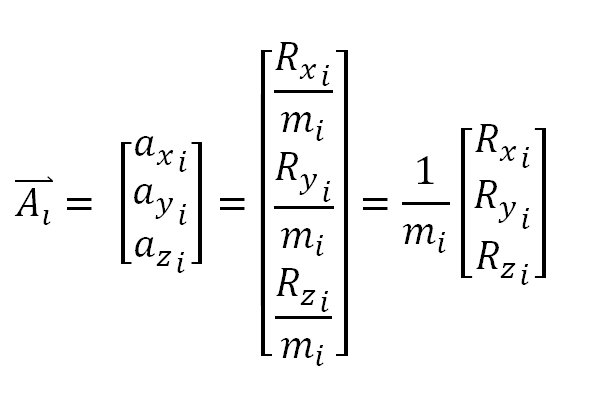The velocity vector can be represented by: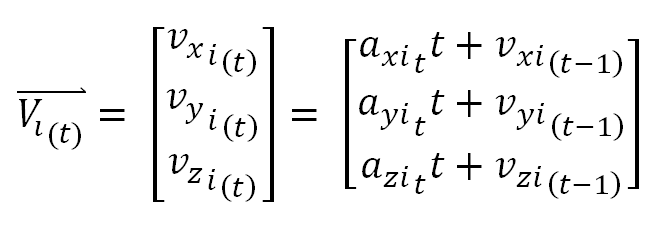# Game

In order to visualize the current method, a game was designed. In this game, there are 10 copies of the Stanford bunny which the user must shoot all of them within three minutes. Two types of guns are provided to the user and he/she must shoot all the bunnies with the lowest shooting rate.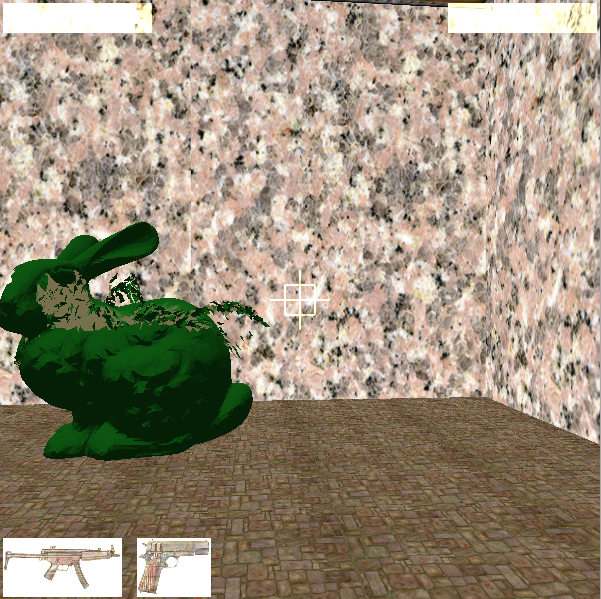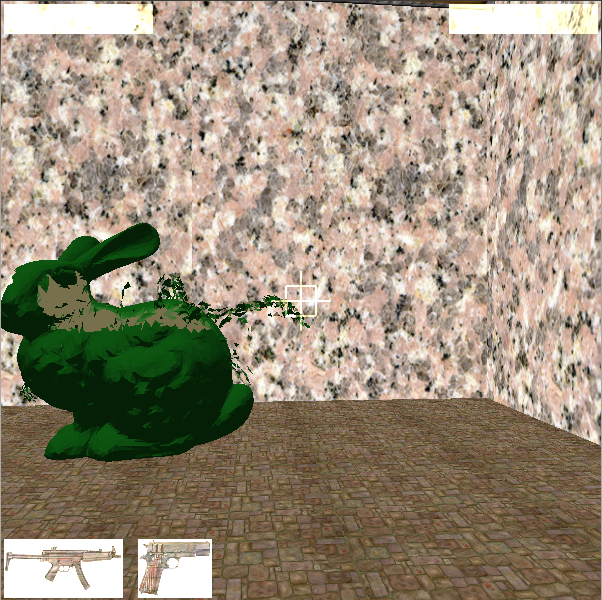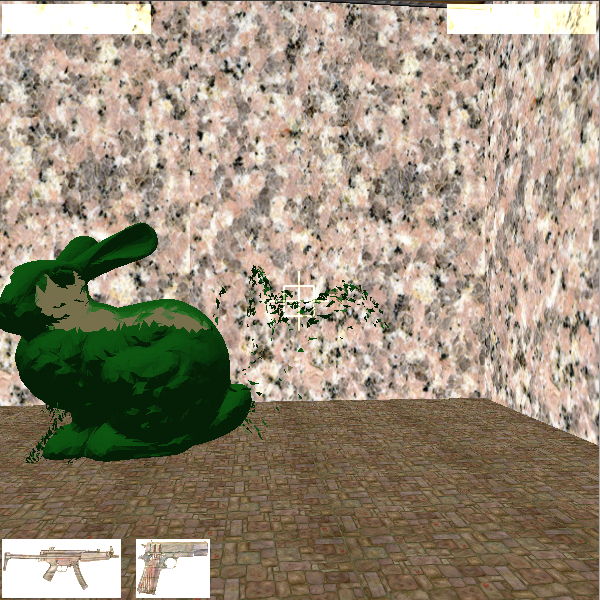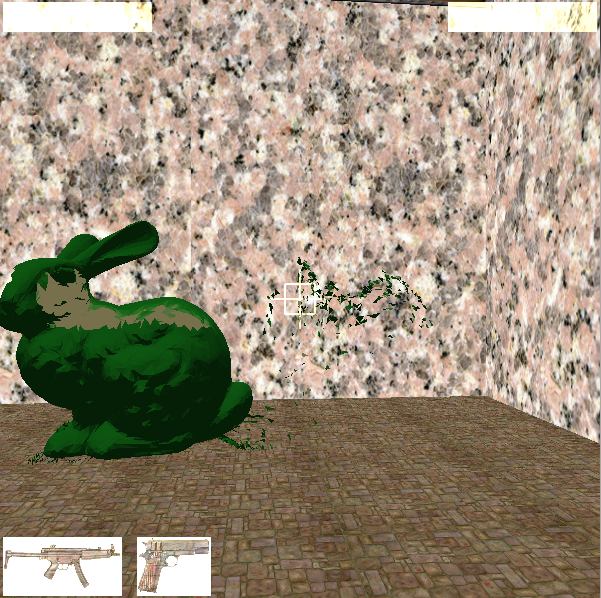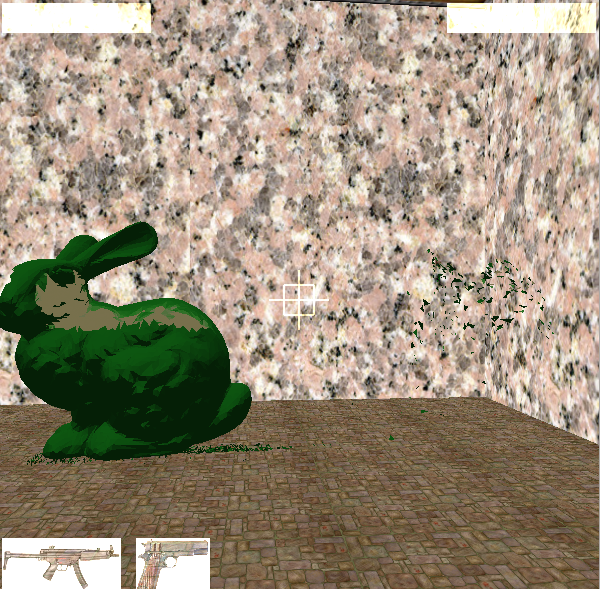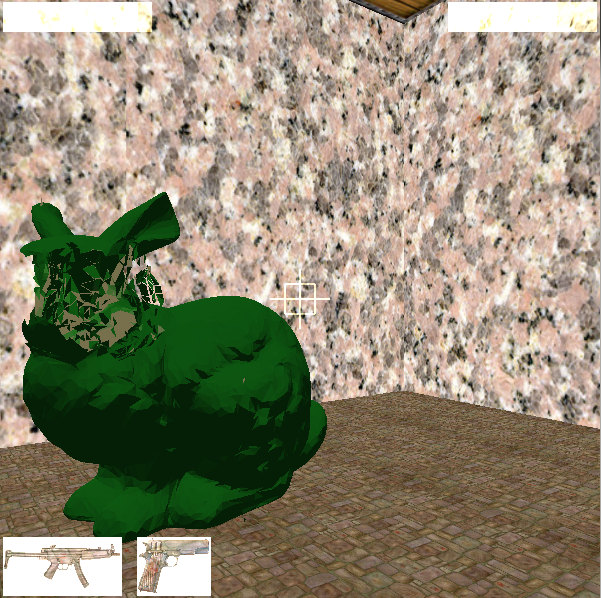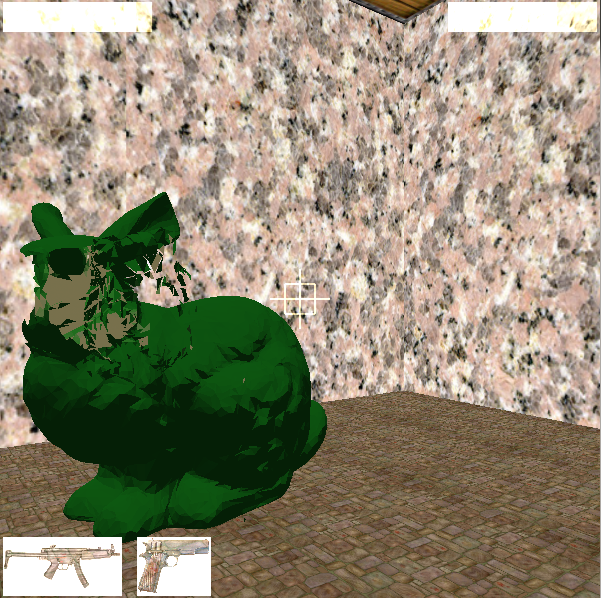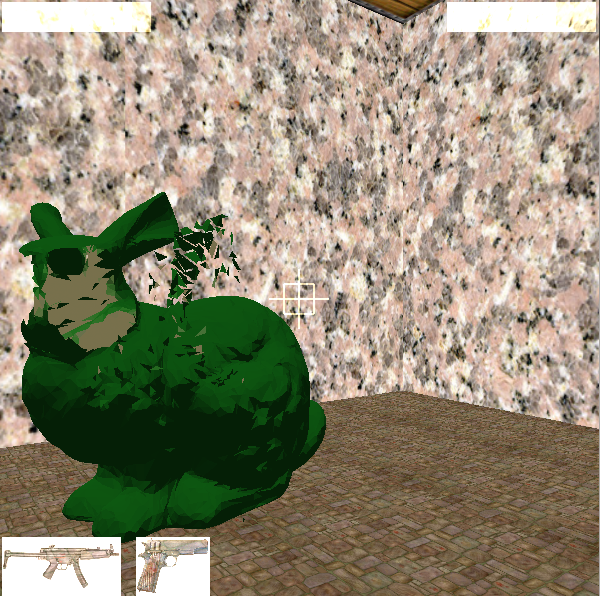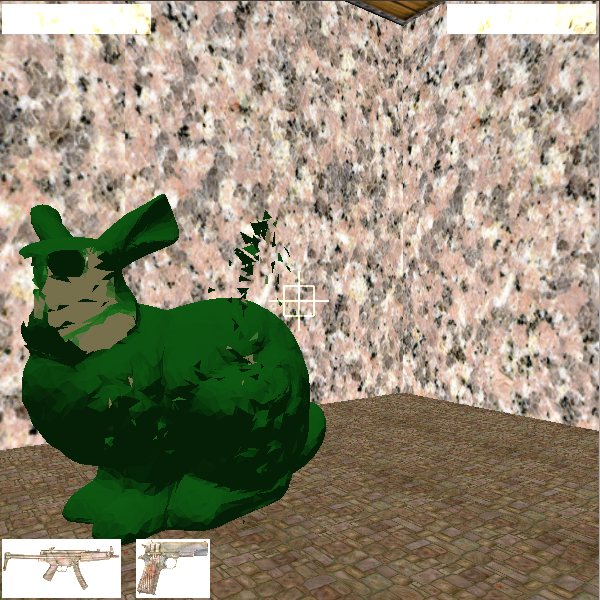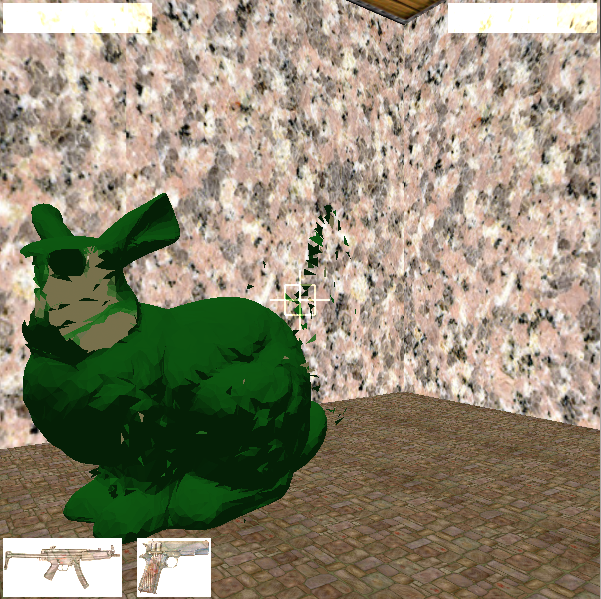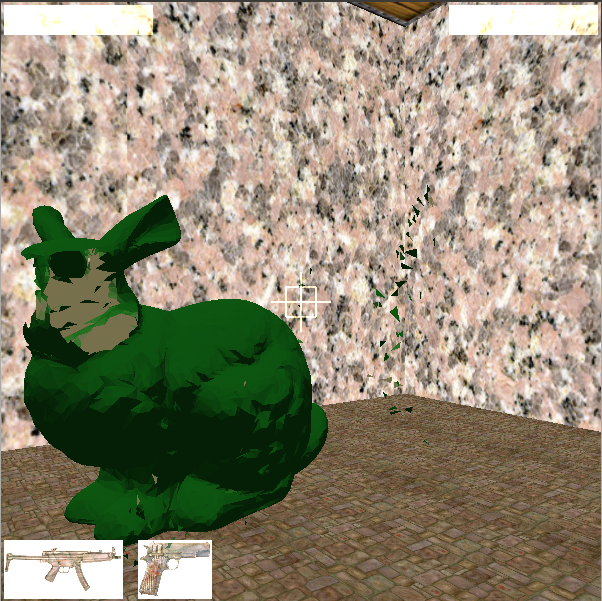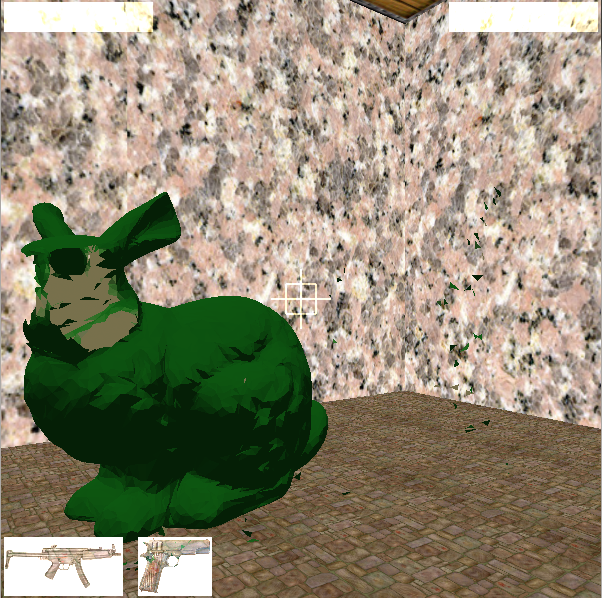Design Downloaded from free CSS templates | free website templates | seodesign.us